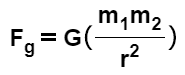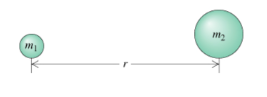# Problem: Learning Goal:To understand Newton's law of universal gravitation and be able to apply it in two-object situations and (collinear) three-object situations; to distinguish between the use of G and g. In the late 1600s, Isaac Newton proposed a rule to quantify the attractive force known as gravity between objects that have mass, such as those shown in the figure. (Figure 1) Newton's law of universal gravitation describes the magnitude of the attractive gravitational force Fg between two objects with masses m1 and m2 aswhere r is the distance between the centers of the two objects and G is the gravitational constant. The gravitational force is attractive, so in the figure it pulls to the right on m1 (toward m2) and toward the left on m2 (toward m1). The gravitational force acting on m1 is equal in magnitude to, but exactly opposite in direction from the gravitational force acting on m2, as required by Newton's third law. The magnitude of both forces is calculated with the equation given above. Consider the earth following its nearly ciruclar orbit (dashed curve) about the sun. The earth has mass mearth = 5.98x1024 kg and the sun has mass msun = 1.99x1030 kg. They are separated, center to center, by r = 93 million miles = 150 million km.What is the magnitude of the graviational force acting on the earth due to the sun?

###### FREE Expert Solution

Gravitational force:

$\overline{){{\mathbf{F}}}_{{\mathbf{g}}}{\mathbf{=}}{\mathbf{G}}{\mathbf{\left(}}\frac{{\mathbf{m}}_{\mathbf{1}}{\mathbf{m}}_{\mathbf{2}}}{{\mathbf{r}}^{\mathbf{2}}}{\mathbf{\right)}}}$

100% (296 ratings)###### Problem Details

Learning Goal:

To understand Newton's law of universal gravitation and be able to apply it in two-object situations and (collinear) three-object situations; to distinguish between the use of G and g.

In the late 1600s, Isaac Newton proposed a rule to quantify the attractive force known as gravity between objects that have mass, such as those shown in the figure. (Figure 1) Newton's law of universal gravitation describes the magnitude of the attractive gravitational force Fg between two objects with masses m1 and m2 aswhere r is the distance between the centers of the two objects and G is the gravitational constant.

The gravitational force is attractive, so in the figure it pulls to the right on m1 (toward m2) and toward the left on m2 (toward m1). The gravitational force acting on m1 is equal in magnitude to, but exactly opposite in direction from the gravitational force acting on m2, as required by Newton's third law. The magnitude of both forces is calculated with the equation given above.Consider the earth following its nearly ciruclar orbit (dashed curve) about the sun. The earth has mass mearth = 5.98x1024 kg and the sun has mass msun = 1.99x1030 kg. They are separated, center to center, by r = 93 million miles = 150 million km.

What is the magnitude of the graviational force acting on the earth due to the sun?